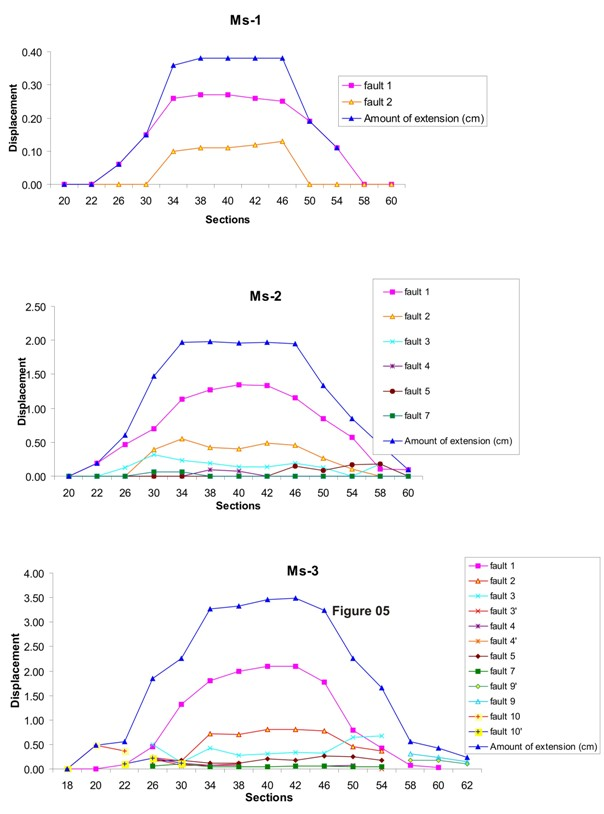## Displacement along the Fault Planes

All these models show a similar pattern for the development of the graben. In all the experiments, the maximum displacement is observed along fault 1 which is the surface expression of low angle blind normal fault.

### First Model

In the first experiment the total displacement is 0.5cm. The first synthetic fault has 0.06cm to 0.27cm displacement on different sections (from 26cm to 54cm sections) while the second fault (antithetic fault) has maximum displacement (0.13cm) in 46cm section. The highest total displacement is 0.40cm observed in the central part of the experiment (40cm-42cm sections).

### Second Model

The second model developed at 2.0cm displacement along a blind normal fault. On fault 1 we measured from 0.11cm (at 22cm section) up to 1.35cm (at 40cm section) of displacement. The displacement along the second and third faults is 0.40cm and 0.15cm, respectively. The maximum displacement is 1.35cm along fault 1 and the total displacement in this model is 2.0cm (corresponding to the imposed one).

### Third Model

The third model has 3.5cm of extension by the moving wall. A deep graben with a number of faults in the central part and the second graben near the moving wall. In this central graben the displacement along first order faults is highest; fault 1 has 0.9 (at section 22cm) to 2.10cm (at section 40cm), fault 2 has 0.16cm (at section 22cm) to 0.81cm (at section 42cm) extension, and fault 3 has 0.13cm (at section 30cm) to 0.67cm (at section 54cm) extension. In the second graben, fault 5 has 0.12cm to 0.26cm and fault 7 has 0.04cm to 0.1cm displacement. The highest displacement along the fault 1 is 2.10cm. In this experiment the total displacement is 3.46cm.

Figure 5. Displacement with respect to the total amount of extensionBinary graphs showing displacement with respect to the total extension (in cm). The X-axis is relative to the dimensions of the models, the Y-axis show the displacement along fault plane. All models exhibit quasi-elliptical shape for slip distribution. Ms-3 has a deepest graben and, accordingly, it has a highest displacement among others.